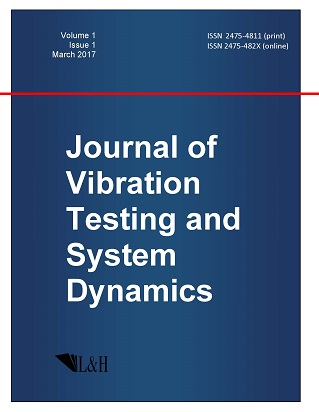ISSN: 2475-4811 (print)
ISSN: 2475-482X (online)
Journal of Vibration Testing and System Dynamics

C. Steve Suh (editor), Pawel Olejnik (editor),

Xianguo Tuo (editor)

Pawel Olejnik (editor)

Lodz University of Technology, Poland

Email: pawel.olejnik@p.lodz.pl

C. Steve Suh (editor)

Texas A&M University, USA

Email: ssuh@tamu.edu

Xiangguo Tuo (editor)

Sichuan University of Science and Engineering, China

Email: tuoxianguo@suse.edu.cn

Branching Rules and Subduced Representations Applied to Lie Point Symmetries of Differential Equations

Journal of Vibration Testing and System Dynamics 7(1) (2023) 5--13 | DOI:10.5890/JVTSD.2023.03.002

R. Campoamor-Stursberg

Instituto de Matem'atica Interdisciplinar IMI-UCM, Plaza de Ciencias 3, E-28040 Madrid, Spain

Abstract

Vector field realizations of Lie algebras in connection with the representation theory and the branching rule problem associated to embeddings of semisimple Lie algebras are considered. This allows to determine if a subalgebra in a given realization corresponds to an irreducible embedding, as well as to determine multiplicities in the branching rules. The invariants of the realizations associated to such embeddings are used to construct (second-order) systems of ordinary differential equations possessing a fixed Lie algebra of point symmetries. It is shown that for any embedding $\mathfrak{g}^{\prime}\subset\mathfrak{g}$ and any faithful representation there exists an integer $k$ such that for any $n\geq k$, systems of order $n$ with exact Lie point symmetry $\mathfrak{g}^{\prime}$ can be constructed.

References

1.   Dickson, L.E. (1924), Differential equations from the group standpoint, Annals of Mathematics, 4, 287-378.
2.   Perelomov, A.M. (1990), Integrable Systems of Classical Mechanics and Lie Algebras, Birkh\"auser: Basel.
3.   Olver, P.J. (1986), Applications of Lie Groups to Differential Equations, Springer: New York.
4.   Ibragimov, N.H. (Ed) (1994), CRC Handbook of Lie Group Analysis of Differential Equations, Vol. 2. Applications in Engineering and Physical Sciences, CRC Press: Boca Raton.
5.   Stephani, H., Kramer, D., MacCallum, M., and Hoenselaers, C. (1980), Exact Solutions of Einstein's Field Equations, Cambridge University Press: Cambridge.
6.   Georgi, H. (1982), Lie Algebras in Particle Physics, Addison-Wesley: Boston.
7.   Barbarin, F., Ragoucy, F., and Sorba, P. (1997), Realization of Lie algebras: application to $\mathfrak{so}(4,2)$ and subalgebras, Communications in Mathematical Physics, 186, 393-411.
8.   Grundland, A. and de Lucas, J. (2019), On the geometry of the Clairin theory of conditional symmetries for higher-order systems of PDEs with applications, Differential Geometry and its Applications, 67, 101557.
9.   Campoamor-Stursberg, R. (2018), Reduction by invariants and projection of linear representations of Lie algebras, Journal of Mathematical Physics, 59, 033502.
10.   Kamke, E. (1961), Differentialgleichungen. L\"osungsmethoden und L\"osungen. Band II, Akademische Verlagsgesellschaft: Leipzig.
11.   McKay, W.G. and Patera, J. (1981), Tables of Dimensions, Indices, and Branching Rules for Representations of Simple Lie Algebras, Marcel Dekker: New York.
12.   Iwahori, N. (1959), On real irreducible representations of Lie algebras, Nagoya Mathematical Journal, 14, 59-83.
13.   Patera, J. and Sankoff, D. (1973), Tables of Branching Rules for Representations of Simple Lie Algebras, Presses de l'Universite de Montreal: Montreal.
14.   King, R.C. (1975), Branching rules for classical Lie groups using tensor and spinor methods, Journal of Physics A: Mathematical and General, 8, 429-449.
15.   Proctor, R.A. (1994), Young tableaux, Gel'fand patterns and branching rules for classical Lie groups, Journal of Algebra, 164, 299-360.
16.   Douglas, J. (1941), Solution of the inverse problem of the calculus of variations, Transactions of the American Mathematical Society, 50, 71-128.
17.   Anderson, I.M. and Duchamp, T.E. (1980), On the existence of global variational principles, American Journal of Mathematics, 102, 781-868.
18.   Anderson, I.M. and Thompson, G. (1992), The Inverse Problem of the Calculus of Variations for Ordinary Differential Equations, Memoirs American Mathematical Society, 473.
19.   Campoamor-Stursberg, R. (2018), An inverse problem in Lagrangian dynamics based on the preservation of symmetry groups: application to systems with a position-dependent-mass, Acta Mechanica, 229, 211-229.